# Introduction

This tutorial provides a comprehensive introduction to a modeling and simulation workflow in Pumas. This tutorial will not get into the details of Pumas specifics, but instead provide a narrative on the lines of a regular workflow in our day to day work, with brevity where required to allow a broad overview. Wherever possible, cross-references will be provided to documentation and detailed examples that provide deeper insight into a particular topic.

As part of this workflow in part-1 you will be introduced to various aspects such as

1. Data wrangling in Julia

2. Exploratory analysis in Julia

3. Continuos data non-linear mixed effects modeling in Pumas

4. Model comparison routines, post-processing, validation etc.

Part-2 of this tutorial will dive into discrete data modeling where the exposure from the PK model developed here will drive the outcome.

## The Study and Design

CTMNopain is a novel anti-inflammatory agent under preliminary investigation. A dose-ranging trial was conducted comparing placebo with 3 doses of CTMNopain (5mg, 20mg and 80 mg QD). The maximum tolerated dose is 160 mg per day. Plasma concentrations (mg/L) of the drug were measured at 0, 0.5, 1, 1.5, 2, 2.5, 3-8 hours.

Pain score (0=no pain, 1=mild, 2=moderate, 3=severe) were obtained at time points when plasma concentration was collected. A pain score of 2 or more is considered as no pain relief.

The subjects can request for remedication if pain relief is not achieved after 2 hours post dose. Some subjects had remedication before 2 hours if they were not able to bear the pain. The time to remedication and the remedication status is available for subjects.

The pharmacokinetic dataset can be viewed here, and later in the tutorial, we show you how to download for use. The remedication dataset with binary, ordinal and censored data will be used in part-2 of the tutorial (to be released shortly).

## Setup

Two libraries provide the workhorse functionality in the Pumas ecosystem that we will load:

using Pumas
using PumasUtilities


The above two packages come in-built with your download and you don't need to specifically add them. You can start using them directly.

using GLM: lm, @formula
using Weave
using Random
using CSV
using HTTP
using Chain
using Latexify
using CairoMakie


If you get a message that any of these ancillary packages are not available in your system, please add them as follows e.g.

using Pkg


### Data Wrangling

We start by reading in the dataset and making some quick summaries.

Note: As a general convention during this example, dataframes will be referred by ending with the name of the variable with df and the Population version of that dataframe will be without the df to avoid confusion.

f = CSV.File(HTTP.get("http://bit.ly/painremed").body,
missingstrings=["", "NA", "."])

pkpain_df = DataFrame(f)


Let's filter out the placebo data as we don't need that for the PK analysis.

pkpain_noplb_df = filter(x -> !(occursin.("Placebo", x.Dose)), pkpain_df)
first(pkpain_noplb_df, 10)


10 rows × 7 columns

SubjectTimeConcPainReliefPainScoreRemedStatusDose
Int64Float64Float64Int64Int64Int64String
110.00.003120 mg
210.51.1557811020 mg
311.01.3721110020 mg
411.51.3005810020 mg
512.01.1919511020 mg
612.51.1360211020 mg
713.00.87322410020 mg
814.00.73996311020 mg
915.00.60014302020 mg
1016.00.42562411020 mg

## Analysis

### Non-compartmental analysis

Let's begin by performing a quick NCA of the concentration time profiles and view the exposure changes across doses. The input data specification for NCA analysis requires the presence of a route column and an amount column that specifies the dose. So, let's add that in.

#adding route variable
pkpain_noplb_df[!,:route] .= "ev"
# creating an amt column
pkpain_noplb_df[!,:Dose] .= parse.(Int,  chop.(pkpain_noplb_df.Dose, tail=3))
pkpain_noplb_df[!,:amt] .= ifelse.(pkpain_noplb_df.Time .== 0, pkpain_noplb_df.Dose, missing)


Now, we map the data variables to the read_nca function that prepares the data for NCA analysis.

pkpain_nca = read_nca(pkpain_noplb_df,
id = :Subject,
time = :Time,
amt = :amt,
observations = :Conc,
group = [:Dose],
route = :route)


Now that we mapped the data in, let's visualize the concentration vs time plots for a few individuals. When paginate is set to true, a vector of plots are returned and below we display the first element with 9 individuals.

f = observations_vs_time(pkpain_nca,
paginate = true,

axis = (xlabel = "Time (hr)",
ylabel = "CTMNoPain Concentration (ng/mL)"),
facet = (combinelabels =true,)
)
f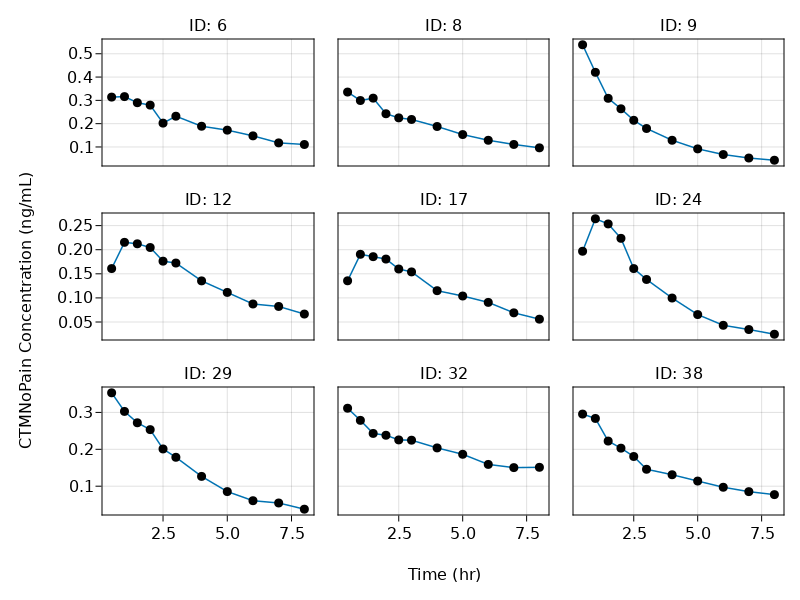or you can view the summary curves by dose group as passed in to the group argument in read_nca

f = summary_observations_vs_time(
pkpain_nca,
figure = (
fontsize = 22,
resolution = (800,1000),
),
color = "black", linewidth = 3,
axis = (
xlabel = "Time (hr)",
ylabel = "CTMX Concentration (μg/mL)",
),
facet = (combinelabels = true,

)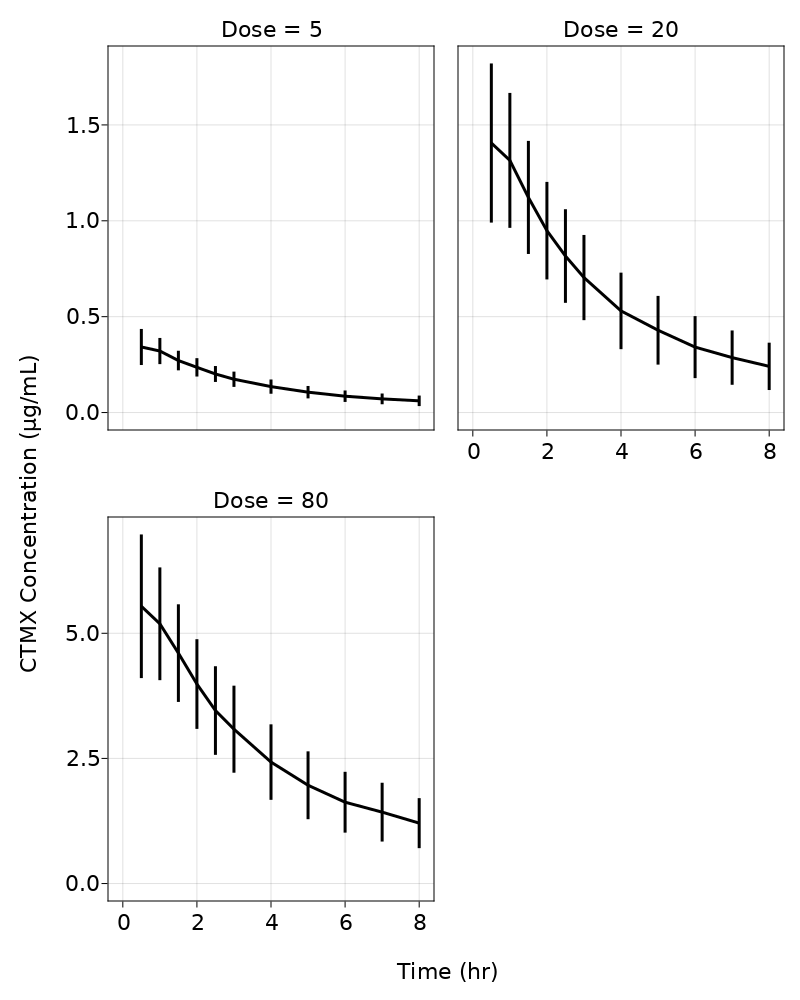A full NCA Report is now obtained for completeness purposes using the run_nca function, but later we will only extract a couple of key metrics of interest.

pk_nca = run_nca(pkpain_nca, sigdig=3)
first(pk_nca.reportdf, 10)


10 rows × 40 columns (omitted printing of 31 columns)

idDosedoseamttlagtmaxcmaxtlastclastclast_pred
Int64Int64Int64Float64Float64Float64Float64Float64Float64
16550.01.00.3161158.00.1106160.107079
28550.00.50.3356498.00.09622410.0960789
39550.00.50.5385668.00.04308120.0387015
412550.01.00.2153028.00.06649530.0653076
517550.01.00.1904828.00.05588570.0553108
624550.01.00.2641858.00.02463950.0236468
729550.00.50.3531268.00.03768470.0366889
832550.00.50.3113698.00.1511860.143299
938550.00.50.2954938.00.07715990.0758813
1040550.00.50.448798.00.06501220.0645857

We can look at the NCA fits for some subjects.

f = subject_fits(pk_nca,
paginate = true,
axis  = (xlabel = "Time (hr)",
ylabel = "CTMX Concentration (μg/mL)"),
facet = (combinelabels = true,
)


As CTMNopain's effect maybe mainly related to maximum concentration (cmax) or area under the curve (auc), we present some summary statistics using the summarize function from NCA.

strata = [:Dose]
parms = [:cmax, :aucinf_obs]
output = summarize(pk_nca.reportdf; stratify_by = strata, parameters = parms)


6 rows × 9 columns (omitted printing of 2 columns)

DoseparametersextremageomeangeomeanCVgeostdmean
Int64StringTuple…Float64Float64Float64Float64
15aucinf_obs(0.9137, 3.39947)1.5339786.69641.329891.59819
25cmax(0.190482, 0.538566)0.345132374.6211.292940.356087
320aucinf_obs(2.77495, 14.1139)6.0197523.48211.413566.3764
420cmax(0.933113, 2.70061)1.4339988.07871.263041.47354
580aucinf_obs(13.7102, 49.122)28.29734.740711.3414929.5023
680cmax(3.29685, 8.47195)5.6412522.29481.257715.78671

The statistics printed above are the default, but you can pass in your own statistics using the stats = [] argument to the summarize function.

We can look at a few parameter distribution plots.

f = parameters_vs_group(pk_nca,
parameter = :cmax,
axis = (xlabel = "Dose (mg)",
ylabel = "Cₘₐₓ (ng/mL)"),
figure = (fontsize = 18, ))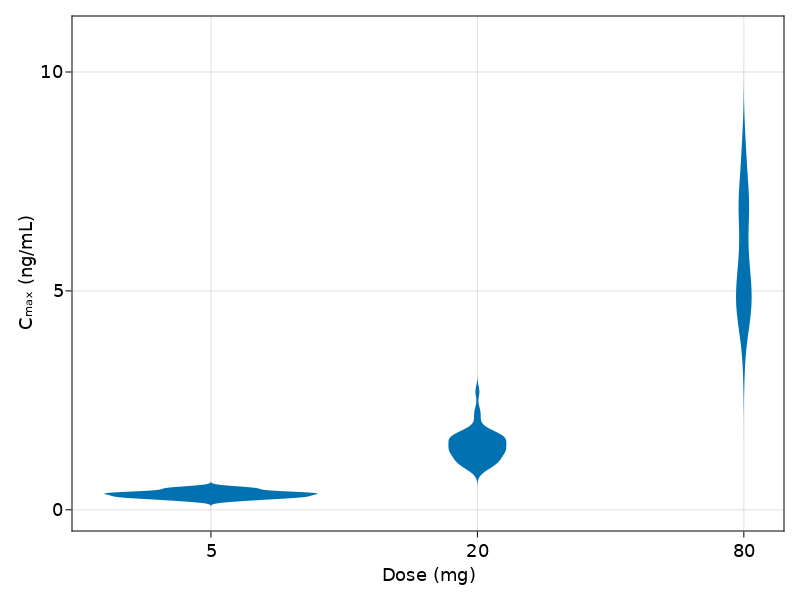Dose normalized PK parameters, cmax and aucinf were essentially dose proportional between for 5 mg, 20 mg and 80 mg doses. You can perform a simple regression to check the impact of dose on cmax

dp = lm(@formula(cmax~Dose), pk_nca.reportdf)

StatsModels.TableRegressionModel{GLM.LinearModel{GLM.LmResp{Vector{Float64}
}, GLM.DensePredChol{Float64, LinearAlgebra.CholeskyPivoted{Float64, Matrix
{Float64}}}}, Matrix{Float64}}

cmax ~ 1 + Dose

Coefficients:
───────────────────────────────────────────────────────────────────────────
Coef.  Std. Error      t  Pr(>|t|)   Lower 95%  Upper 95%
───────────────────────────────────────────────────────────────────────────
(Intercept)  0.00970894   0.105954    0.09    0.9271  -0.200109   0.219527
Dose         0.0722591    0.0022214  32.53    <1e-60   0.0678601  0.0766581
───────────────────────────────────────────────────────────────────────────


Based on visual inspection of the concentration time profiles as seen earlier, CTMNopain exhibited monophasic decline, and perhaps a one compartment model best fits the PK data.

### Pharmacokinetic modeling

As seen from the plots above, the concentrations decline monoexponentially. We will evaluate both one and two compartment structural models to assess best fit. Further, different residual error models will also be tested.

We will use the results from NCA to provide us good initial estimates. The mean clearance is 3.29, the mean volume is 16.45 and a good initial estimate for absorption rate as obtained by "$\frac{0.693}{\frac{tmax}{4}}$" is 3.85

#### Data preparation for modeling

PumasNDF requires the presence of evid and cmt columns in the dataset.

pkpain_noplb_df[!, :evid] .= ifelse.(pkpain_noplb_df.Time .== 0, 1, 0)
pkpain_noplb_df[!, :cmt] .= ifelse.(pkpain_noplb_df.Time .== 0, 1, 2)
pkpain_noplb_df[!, :cmt2] .= 1 # for zero order absorption


Further, observations at time of dosing, i.e., when evid = 1 have to be missing

pkpain_noplb_df[!, :Conc] .= ifelse.(pkpain_noplb_df.evid .== 1, missing, pkpain_noplb_df.Conc)


The dataframe will now be converted to a Population using read_pumas. Note that both observations and covariates are required to be an array even if it is one element.

pkpain_noplb = read_pumas(pkpain_noplb_df,
id           = :Subject,
time         = :Time,
amt          = :amt,
observations = [:Conc],
covariates   = [:Dose],
evid         = :evid,
cmt          = :cmt)


Now that the data is transformed to a Population of subjects, we can explore different models.

#### One-compartment model

pk_1cmp = @model begin
desc = "One Compartment Model"
timeu = u"hr"
end
@param begin
"Clearance (L/hr)"
tvcl ∈ RealDomain(lower = 0, init = 3.2)
"Volume (L)"
tvv  ∈ RealDomain(lower = 0, init = 16.4)
"Absorption rate constant (h-1)"
tvka ∈ RealDomain(lower = 0, init = 3.8)
"""
- ΩCL
- ΩVc
- ΩKa
"""
Ω    ∈ PDiagDomain(init = [0.04,0.04,0.04])
"Proportional RUV"
σ_p  ∈ RealDomain(lower = 0.0001, init = 0.2)
end
@random begin
η ~ MvNormal(Ω)
end
@covariates begin
"Dose (mg)"
Dose
end
@pre begin
CL = tvcl * exp(η)
Vc = tvv * exp(η)
Ka = tvka * exp(η)
end
@dynamics Depots1Central1
@derived begin
cp := @. Central/Vc
"""
CTMx Concentration (ng/mL)
"""
Conc ~ @. Normal(cp, abs(cp)*σ_p)
end
end

PumasModel
Parameters: tvcl, tvv, tvka, Ω, σ_p
Random effects: η
Covariates: Dose
Dynamical variables: Depot, Central
Derived: Conc
Observed: Conc


Before going to fit the model, let's evaluate some helpful steps via simulation to check appropriateness of data and model

#zero out the random effects
etas = zero_randeffs(pk_1cmp,
init_params(pk_1cmp),
pkpain_noplb)


Above, we are generating a vector of η's of the same lenght as the number of subjects to zero out the random effects. We do this as we are evaluating the trajectories of the concentrations at the initial set of parameters at a population level. Other helper functions here are sample_randeffs and init_randeffs. Please refer to the documentation.

simpk = simobs(pk_1cmp,
pkpain_noplb,
init_params(pk_1cmp), etas)
f = sim_plot(pk_1cmp,
simpk, observations =[:Conc],
figure = (fontsize = 18, ),
axis = (xlabel = "Time (hr)",
ylabel = "Observed/Predicted \n CTMx Concentration (ng/mL)", ))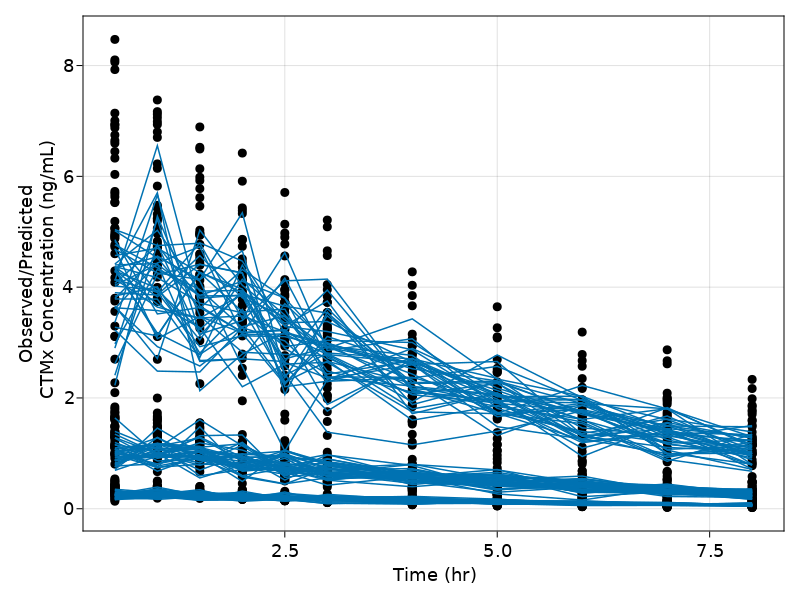Our NCA based initial guess on the parameters seem to work well.

Lets change the initial estimate of a couple of the parameters to evaluate the sensitivity.

pkparam = (init_params(pk_1cmp)..., tvka=2, tvv = 10)

(tvcl = 3.2, tvv = 10, tvka = 2, Ω = [0.04 0.0 0.0; 0.0 0.04 0.0; 0.0 0.0 0
.04], σ_p = 0.2)

simpk = simobs(pk_1cmp,
pkpain_noplb,
pkparam, etas)
f = sim_plot(pk_1cmp,
simpk, observations =[:Conc],
figure = (fontsize = 18, ),
axis = (xlabel = "Time (hr)",
ylabel = "Observed/Predicted \n CTMx Concentration (ng/mL)", ))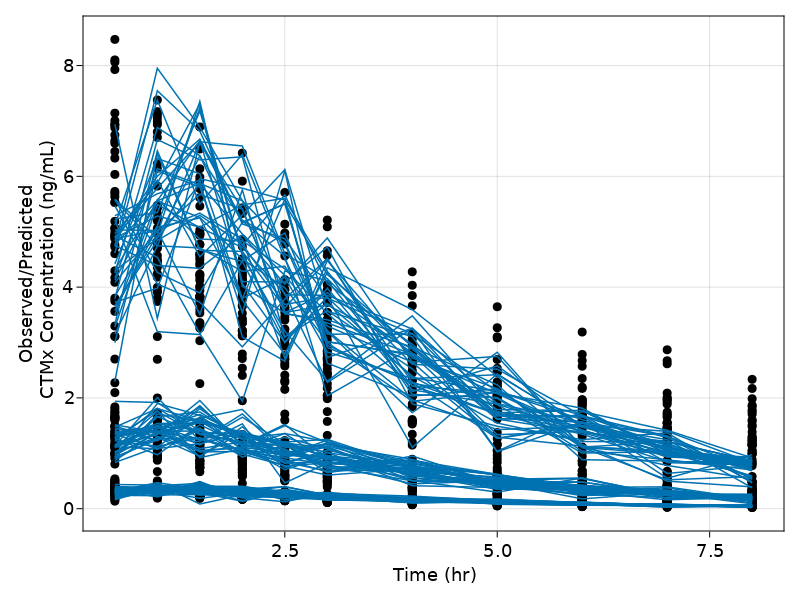Changing the tvka and decreasing the tvv seemed to make an impact and observations go through the simulated lines. In the interactive REPL session, you can use the explore_estimates function to generate an interactive app on the fly that provides you controls and live updating plots. Please check the documentation on creating interactive apps for more details.

To get a quick ballpark estimate of your PK parameters, we can do a NaivePooled analysis. Below we test the NaivePooled approach

##### NaivePooled
pkfit_np = fit(pk_1cmp,
pkpain_noplb,
init_params(pk_1cmp),
Pumas.NaivePooled(),
omegas = (:Ω,))

coefficients_table(pkfit_np)


7 rows × 3 columns

ParameterDescriptionEstimate
StringAbstrac…Float64
1tvclClearance (L/hr)3.01
2tvvVolume (L)14.1
3tvkaAbsorption rate constant (h-1)44.2
4Ω₁,₁ΩCLNaN
5Ω₂,₂ΩVcNaN
6Ω₃,₃ΩKaNaN
7σ_pProportional RUV0.33

The final estimates from the NaivePooled approach seem reasonably close to our initial guess from NCA, except for the tvka parameter. We will stick with our initial guess

One way to be cautious before going into a complete fitting routine is to evaluate the likelihood of the individual subjects given the initial parameter values and see if any subject(s) pops out as unreasonable. There are a few ways of doing this:

• check the loglikelihood subject wise

• check if there any influential subjects

Below, we are basically checking if the initial estimates for any subject are way off that we are unable to compute the initial loglikelihood.

lls = []
for subj in pkpain_noplb
push!(lls,loglikelihood(pk_1cmp,
subj,
pkparam,
Pumas.FOCE()))
end
# the plot below is using native CairoMakie
hist(lls; bins = 10, normalization = :none, color = (:black, 0.5), x_gap = 0)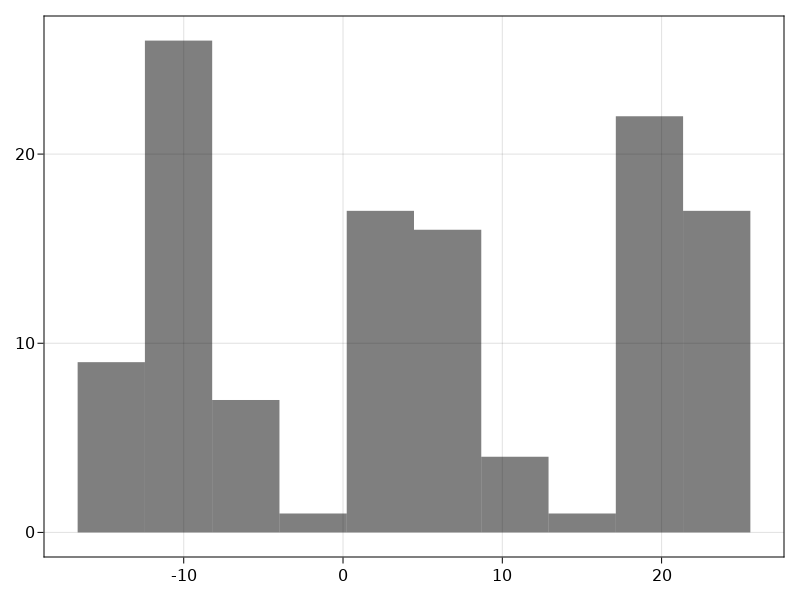The distribution of the loglikelihood's suggest no extreme outliers.

A more convenient way is to use the findinfluential function that provides a list of k top influential subjects by showing the (minus) loglikelihood for each subject. As you can see below, the minus loglikelihood in the range of 16 agrees with the histogram plotted above.

influential_subjects = findinfluential(pk_1cmp,
pkpain_noplb,
pkparam,
Pumas.FOCE())

5-element Vector{Tuple{String, Float64}}:
("148", 16.659658856844857)
("135", 16.648985190076342)
("156", 15.959069556607492)
("159", 15.441218240496486)
("149", 14.715134644119516)


Now that we have a good handle on our data, lets go ahead and fit a population model

pkfit_1cmp = fit(pk_1cmp,
pkpain_noplb,
pkparam,
Pumas.FOCE(),
constantcoef = (tvka = 2,))

infer(pkfit_1cmp)

Asymptotic inference results using sandwich estimator

Successful minimization:                      true

Likelihood approximation:               Pumas.FOCE
Log-likelihood value:                    1231.1881
Number of subjects:                            120
Number of parameters:         Fixed      Optimized
1              6
Observation records:         Active        Missing
Conc:                      1320              0
Total:                     1320              0

-----------------------------------------------------------------
Estimate           SE                    95.0% C.I.
-----------------------------------------------------------------
tvcl     3.1642          0.08662         [ 2.9944  ;  3.334  ]
tvv     13.288           0.27482         [12.749   ; 13.827  ]
tvka     2.0             NaN             [  NaN    ;   NaN     ]
Ω₁,₁     0.084939        0.011021        [ 0.063338;  0.10654]
Ω₂,₂     0.048566        0.0063493       [ 0.036121;  0.06101]
Ω₃,₃     5.5812          1.2198          [ 3.1905  ;  7.9719 ]
σ_p      0.10093         0.0057197       [ 0.089719;  0.11214]
-----------------------------------------------------------------


Notice that tvka is fixed to 2 as we don't have a lot of information before tmax. From the results above, we see that the parameter precision for this model is reasonable.

Just to be sure, let's fit a 2-compartment model and evaluate

pk_2cmp = @model begin
@param begin
"Clearance (L/hr)"
tvcl ∈ RealDomain(lower = 0, init = 3.2)
"Central Volume (L)"
tvv  ∈ RealDomain(lower = 0, init = 16.4)
"Peripheral Volume (L)"
tvvp ∈ RealDomain(lower = 0, init = 10)
"Distributional Clearance (L/hr)"
tvq  ∈ RealDomain(lower = 0, init = 2)
"Absorption rate constant (h-1)"
tvka ∈ RealDomain(lower = 0, init = 1.3)
"""
- ΩCL
- ΩVc
- ΩKa
- ΩVp
- ΩQ
"""
Ω    ∈ PDiagDomain(init = [0.04,0.04,0.04, 0.04, 0.04])
"Proportional RUV"
σ_p  ∈ RealDomain(lower = 0.0001, init = 0.2)
end
@random begin
η ~ MvNormal(Ω)
end
@covariates begin
"Dose (mg)"
Dose
end
@pre begin
CL = tvcl * exp(η)
Vc = tvv *  exp(η)
Ka = tvka * exp(η)
Vp = tvvp * exp(η)
Q = tvq * exp(η)
end
@dynamics Depots1Central1Periph1
@derived begin
cp := @. Central/Vc
"""
CTMx Concentration (ng/mL)
"""
Conc ~ @. Normal(cp, cp*σ_p)
end
end

PumasModel
Parameters: tvcl, tvv, tvvp, tvq, tvka, Ω, σ_p
Random effects: η
Covariates: Dose
Dynamical variables: Depot, Central, Peripheral
Derived: Conc
Observed: Conc

pkfit_2cmp = fit(pk_2cmp,
pkpain_noplb,
init_params(pk_2cmp),
Pumas.FOCE(),
constantcoef = (tvka = 2,))


The 2 compartment model has a much lower objective function compared to the 1 compartment. Lets compare the estimates from the 2 models using the compare_estimate function.

compare_estimates(;pkfit_1cmp, pkfit_2cmp)


11 rows × 3 columns

parameterpkfit_1cmppkfit_2cmp
StringFloat64?Float64?
1tvcl3.164192.81378
2tvv13.288111.0046
3tvka2.02.0
4Ω₁,₁0.08493890.102669
5Ω₂,₂0.04856590.0607756
6Ω₃,₃5.581171.20116
7σ_p0.1009290.0484049
8tvvpmissing5.53997
9tvqmissing1.51591
10Ω₄,₄missing0.423493
11Ω₅,₅missing0.244732

We perform a likelihood ratio test to compare the two nested models. The test statistic and the P-value clearly indicate that a 2 compartment model is better.

lrtest(pkfit_1cmp, pkfit_2cmp)

Statistic:          1200.0
Degrees of freedom:      4
P-value:               0.0


We should also compare the other metrics and statistics, such ηshrinkage, ϵshrinkage, aic, bic using the metrics_table function.

@chain metrics_table(pkfit_2cmp) begin
leftjoin(metrics_table(pkfit_1cmp), on = :Metric, makeunique = true)
rename!(:Value => :pk2cmp, :Value_1 => :pk1cmp)
end


11 rows × 3 columns

Metricpk2cmppk1cmp
StringFloat64Float64?
1Estimation Time4.31.91
2LogLikelihood (LL)1830.01230.0
3-2LL-3660.0-2460.0
4AIC-3640.0-2450.0
5BIC-3590.0-2420.0
6(η-shrinkage) η₁0.03670.0157
7(η-shrinkage) η₂0.04690.0402
8(η-shrinkage) η₃0.5160.733
9(ϵ-shrinkage) Conc0.1850.105
10(η-shrinkage) η₄0.287missing
11(η-shrinkage) η₅0.154missing

We next generate some goodness of fit plots to compare which model is performing better. To do this, we first inspect the diagnostics of our model fit.

res_inspect_1cmp = inspect(pkfit_1cmp)
res_inspect_2cmp = inspect(pkfit_2cmp)

gof_1cmp = goodness_of_fit(res_inspect_1cmp,
figure = (fontsize = 12,))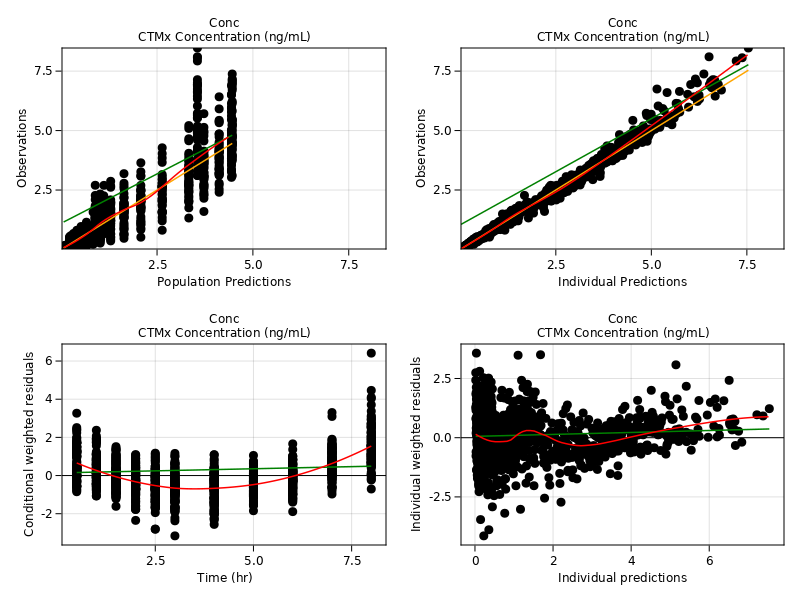gof_2cmp = goodness_of_fit(res_inspect_2cmp,
figure = (fontsize = 12,))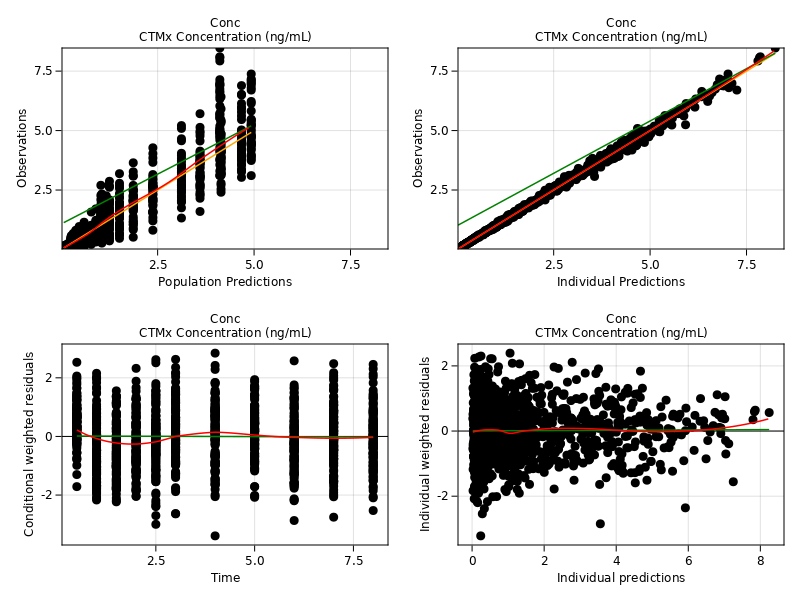These plots clearly indicate that the 2 compartment model is a better fit compared to the one compartment model. In an interactive REPL session, you can use the evaluate_diagnostics(;fittemodel1, fittemodel2) syntax to fire up an app that allows you to compare 2 model fits. You can find more about this function in the documentation.

We can look at selected sample of individual plots.

f = subject_fits(res_inspect_2cmp,
separate = true,
paginate = true,
facet = (combinelabels = true, ),
figure = (fontsize = 18, ),
axis = (xlabel = "Time (hr)",
ylabel = "CTMx Concentration (ng/mL)", ),
legend = (merge = true, unique = true))


We look at random set of 4 individuals here by indexing into generated plot

f